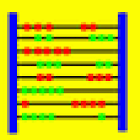Abakus-Func

All Android applications categories

All Android games categories# Abakus-Func

by: 0 0

## Screenshots

Description

Abakus-Func is a computer algebra program which performs symbolic calculations for mathematical functions.

The current Android version 1.0 contains the following features:

- Calculate function values
- Determine exact zeros, singularities, extremal points, and turn points
- Calculate symbolic limits
- Graphical representation (2D and 3D).

As an example, Abakus-Func yields the following exact results for the function f(x) = x/(x+1)^2+2.
Zeros: x = -2, x = -1/2;
Singularities: x = -1;
Extremal points: Maximum (1,9/4);
Turn points: (2,20/9).

You can easily check the symbolic results by viewing the plot of the function. Several functions can be displayed simultaneously within the graphical window. You can select different colors for the individual functions. For functions with two variables, Abakus-Func generates a 3D-like graphical representation.

Moreover Abakus-Func contains a special editor for mathematical formulas. So you can enter even complex expressions very fast.